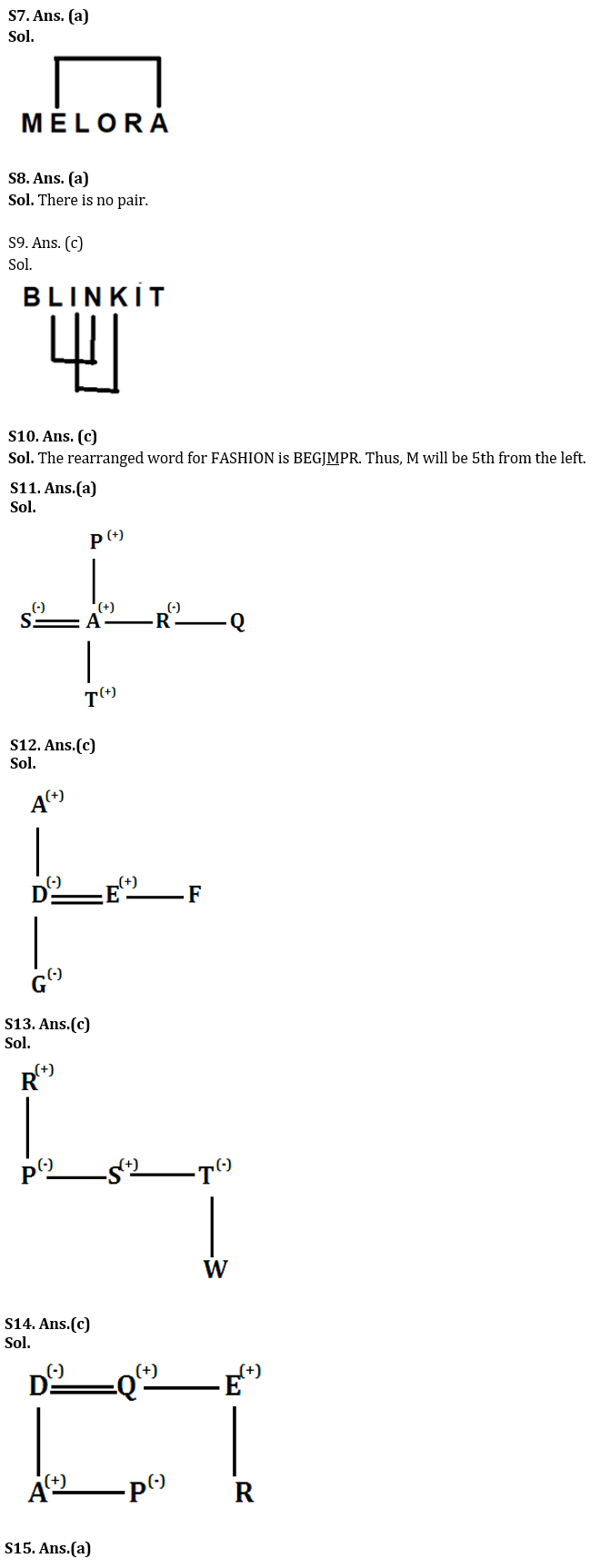# RBI Grade B Phase 1 Reasoning Quiz 2023 -12 March

Topic – Practice Set

Q1. If it is possible to form a meaningful word from the first, third, fifth, seventh and eleventh letters of the word “EXASPERATION”, what would be the first letter of that meaningful word? If you can form more than one such meaningful word, your answer is ‘P’. If no such meaningful word is formed, ‘X’ is your answer.
(a) W
(b) A
(c) P
(Do
(E) X

Q2. If it is possible to form a meaningful word from the 3rd, 7th, 8th, 9th and 11th letters of the word “surrounding”, what would be the last letter of the meaningful word? If you can form more than one such meaningful word, your answer is ‘P’. If no such meaningful word is formed, ‘X’ is your answer.
(a) W
(b) d
(c) I
(d) g
(E) X

Q3. If it is possible to form a meaningful word from the 2nd, 5th, 6th, 8th, 9th, 11th and 12th letters of the word “LOBORIUSNESS”, what would be the 6th letter of the meaningful word? If you can form more than one such meaningful word, your answer is ‘Y’. If no such meaningful word is formed, ‘X’ is your answer.
(a) S
(b) U
(c) I
(d) Y
(E) X

Q4. If all the consonants in the word “SEPTEMBER” are changed to their preceding letter and vowels are changed to their following letter, how many vowels will the newly rearranged word have?
(a) Two
(b) Three
(c) Four
(d) Five
(e) None of the above

Q5. If all the letters of the word “Visakhapatnam” are arranged in reverse alphabetical order from right to left, which of the following would be the correct middle letter?
(is
(b) K
(c) I
(D H
(e) None of these

Q6. If the letters in the odd positions of the word “paralysis” are interchanged with their next letters, which of the following letters will be the correct middle letter?
(is
(b) A
(c) Y
(d) T
(e) None of these

Q7. How many pairs of letters are there in the word “MELORA” that contain letters in a series of letters (both forward and backward)?
(a) One
(b) Two
(c) Three
(d) Four
(e) None of the above

Q8. How many pairs of letters are there in the word “SMYTTEN” that have the same number of letters in a series of letters (both forward and backward)?
(a) None
(b) one
(c) Two
(d) Three
(e) Four
Q9. How many pairs of letters are there in the word “BLINKIT” that have the same number of letters in the letter series (both forward and backward)?
(a) None
(b) one
(c) Two
(d) Three
(e) Four
Q10. If each vowel in the word ‘FASHION’ is changed to its next letter and each consonant is changed to its preceding letter according to the English alphabet, the letters thus formed are arranged alphabetically from left to right, which of the following would be? Fifth letter from the left?
(a) P
(b) g
(cm
(d) J
(e) None of these

Directions (11-14): Each of these questions is based on the following information:
(i) A % B means A is daughter of B.
(ii) A @ B means A is mother of B.
(iii) A \$ B means A is father of B.
(iv) A * B means A is son of B.
(v) A © B means A is brother of B.

Q11. Which of the following relationships shows that S is sister-in-law of Q?
(a) S @ T * A © R % P \$ Q
(b) S% A @ T * R © Q \$ P
(c) T@S © R\$ Q % P
(d) R % S\$ P * Q
(e) None of these

Q12. Who among the following is the niece of F in this relation- ‘A\$D @ G % E © F’?
(a) A
(b) d
(c) G
(d) e
(e) Cannot be determined

Q13. If the statement ‘P % R\$ S © T@W’ is true, which of the following is true?
(a) P is brother of T.
(b) S is daughter of R.
(c) P is aunt of W.
(d) R. This is W’s grandmother.
(e) None is true.

Q14. If the statement ‘A * D @ P % Q © E \$ R’ is true, then which of the following is false?
(a) E is uncle of A.
(b) P is daughter of D.
(c) Q is R’s aunt.
(d) E is brother-in-law of D.
(e) All are true.

Q15. Pointing to a man on stage, Rita said, “He is my husband’s wife’s daughter’s brother. How is the man on stage related to Rita?
(a) Son
(b) Husband
(c) Cousin
(d) Nephew
(e) Nice

Solution

S1. A. (d)
Sol. 1st, 3rd, 5th, 7th and 11th letters of the given word are E, A, P, R, O. Hence the word OPERA can be formed. So ‘O’ will be the first letter of the word.

S2. A. (b)
Sol. The 3rd, 7th, 8th, 9th and 11th letters of the given word are R, N, D, I and G. Hence the word GRIND can be coined. So the last letter of the word is ‘D’.

S3. A. (b)
Sol. 2nd, 5th, 6th, 8th, 9th, 11th and 12th letters of the given word are O, R, I, U, S, E and S. So the word formed is SERIOUS. So the 6th letter of this word is ‘U’.

S4. A. (a)
Sol. Consonants are replaced by their preceding letter and vowels are replaced by their following letter i.e. RFOSFLAFQ. The newly introduced word has two vowels. Hence, option (a) is the answer.